2020-02-06 @Atsushi Kawaguchi

The `msma` package provides functions for a matrix decomposition method incorporating sparse and supervised modeling for a multiblock multivariable data analysis.

# Preparation

Install package (as necessary)

``if(!require("msma")) install.packages("msma")``

``library(msma)``
``## Loading required package: mvtnorm``

# Getting started

Simulated multiblock data (list data) by using the function `simdata`. Sample size is 50. The correlation coeficient is 0.8. The numbers of columns for response and predictor can be specified by the argument `Yps` and `Xps`, respectively. The length of vecor represents the number of blocks. That is, response has three blocks with the numbers of columns being 3, 4, and 5 and predictor has one block with the number of columns being 3.

``````dataset0 = simdata(n = 50, rho = 0.8, Yps = c(3, 4, 5), Xps = 3, seed=1)
X0 = dataset0\$X; Y0 = dataset0\$Y ``````

The argument `comp` can specify the number of components. The arguments `lambdaX` and `lambdaY` can specify the regularization parameters for X and Y, respectively.

• One Component
``````fit01 = msma(X0, Y0, comp=1, lambdaX=0.05, lambdaY=1:3)
fit01``````
``````## Call:
## msma.default(X = X0, Y = Y0, comp = 1, lambdaX = 0.05, lambdaY = 1:3)
##
## Numbers of non-zeros for X:
##        comp1
## block1     3
##
## Numbers of non-zeros for X super:
## comp1
##     1
##
## Numbers of non-zeros for Y:
##        comp1
## block1     1
## block2     1
## block3     1
##
## Numbers of non-zeros for Y super:
## comp1
##     3``````

The `plot` function is available. In default setting, the block weights are displayed as a barplot.

``plot(fit01)``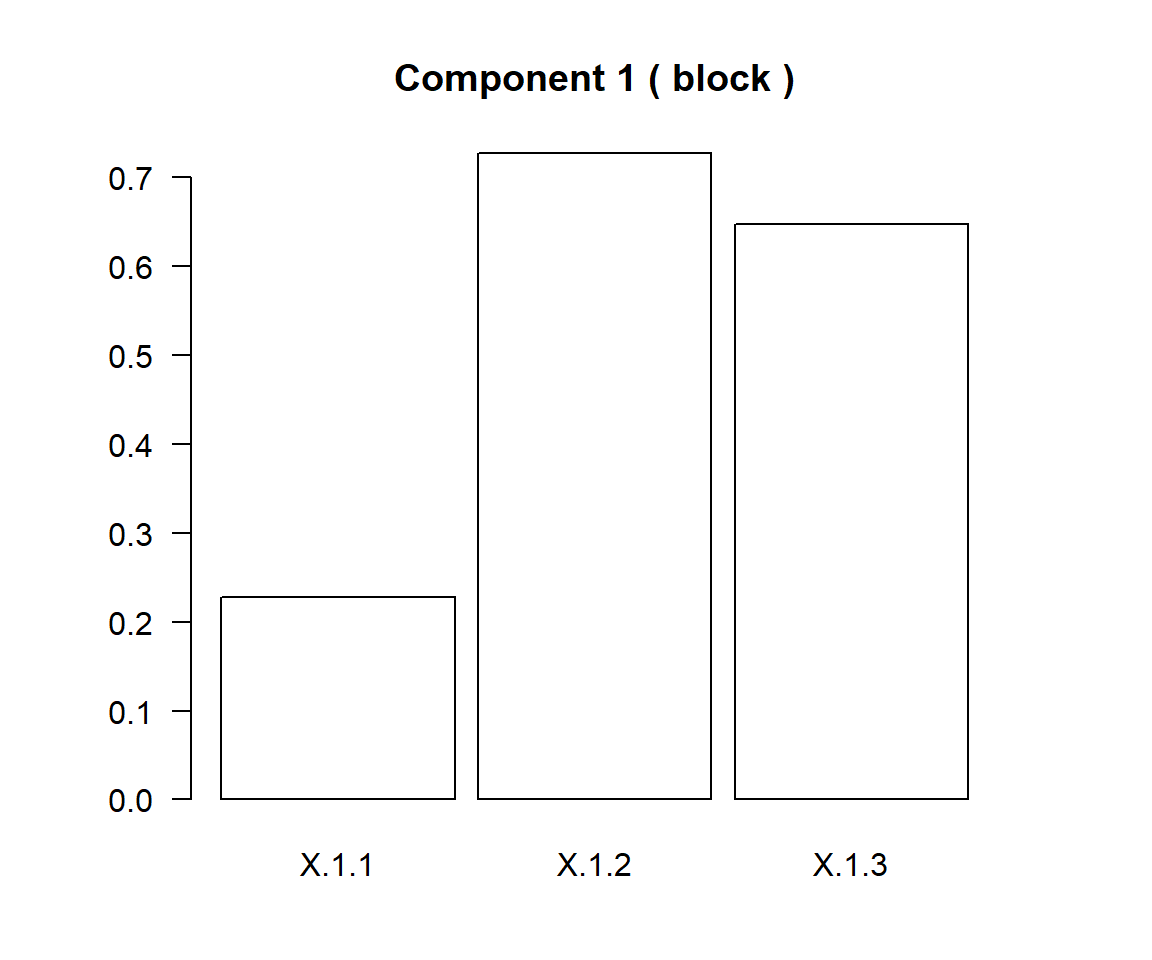• Two Component
``````fit02 = msma(X0, Y0, comp=2, lambdaX=0.03, lambdaY=0.01*(1:3))
fit02``````
``````## Call:
## msma.default(X = X0, Y = Y0, comp = 2, lambdaX = 0.03, lambdaY = 0.01 *
##     (1:3))
##
## Numbers of non-zeros for X:
##        comp1 comp2
## block1     3     3
##
## Numbers of non-zeros for X super:
## comp1 comp2
##     1     1
##
## Numbers of non-zeros for Y:
##        comp1 comp2
## block1     3     3
## block2     4     4
## block3     5     5
##
## Numbers of non-zeros for Y super:
## comp1 comp2
##     3     3``````

# Single Block

Two matrics are prepared by specifying arguments `Yps` and `Xps`.

``````dataset1 = simdata(n = 50, rho = 0.8, Yps = 5, Xps = 5, seed=1)
X1 = dataset1\$X[]; Y1 = dataset1\$Y ``````

## Principal Component Analysis (PCA)

If input is a matrix, a principal component analysis is implemented.

``(fit111 = msma(X1, comp=5))``
``````## Call:
## msma.default(X = X1, comp = 5)
##
## Numbers of non-zeros for X:
##        comp1 comp2 comp3 comp4 comp5
## block1     5     5     5     5     5
##
## Numbers of non-zeros for X super:
## comp1 comp2 comp3 comp4 comp5
##     1     1     1     1     1``````

``fit111\$wbX``
``````## []
##           comp1       comp2       comp3         comp4       comp5
## X.1.1 0.4309622 -0.74170223 -0.03672379  0.1325580413 -0.49520613
## X.1.2 0.4483196  0.31188303  0.63228246  0.5490205405  0.02310504
## X.1.3 0.4601597 -0.19547078 -0.38567734  0.1474129336  0.76129277
## X.1.4 0.4392794  0.55811865 -0.57117598 -0.0006449093 -0.41145448
## X.1.5 0.4566923  0.05386584  0.35196769 -0.8119567864  0.07331836``````

The bar plots of weight vectors are provided by the function `plot`. The component number is specified by the argument `axes`. The plot type is selected by the argument `plottype`.

``````par(mfrow=c(1,2))
plot(fit111, axes = 1, plottype="bar")
plot(fit111, axes = 2, plottype="bar")``````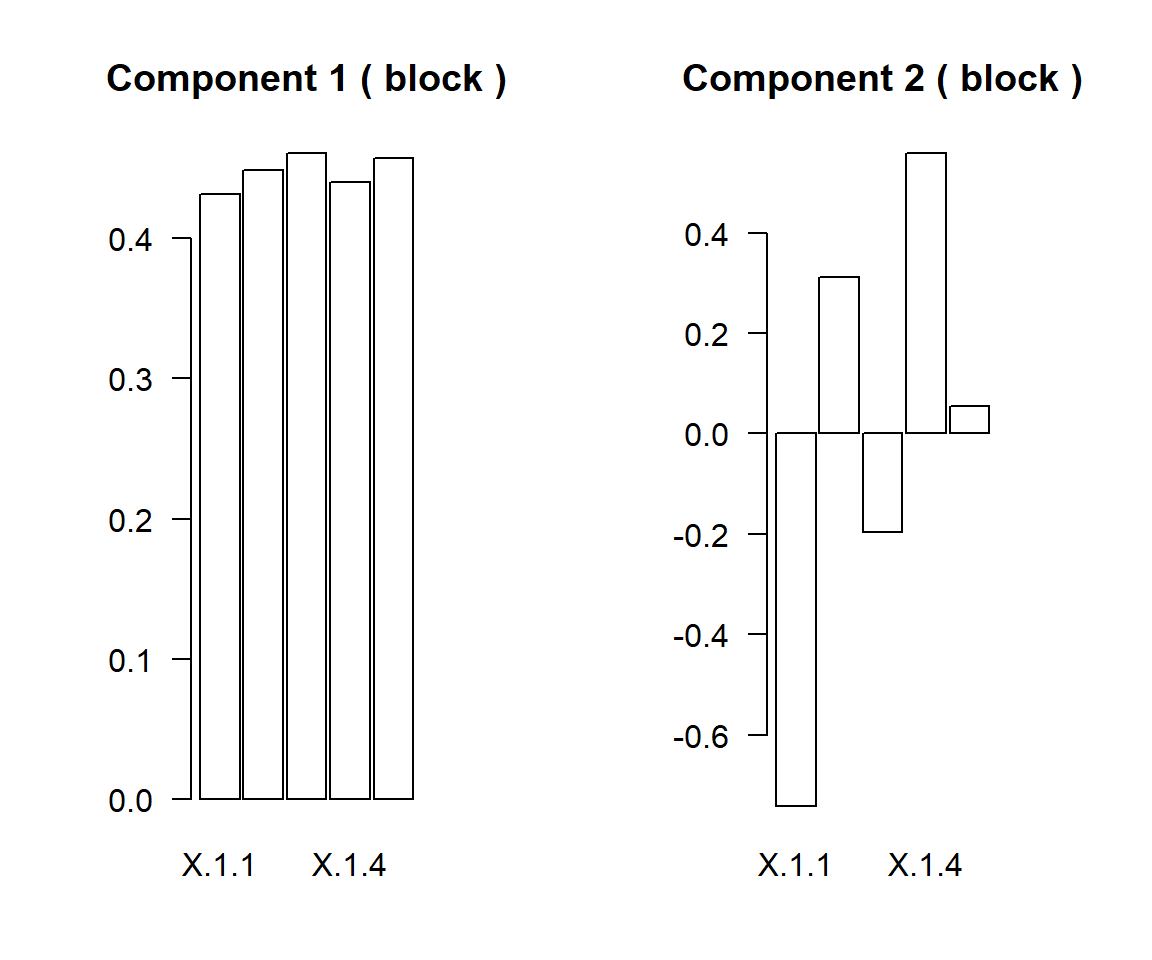The score vectors for first six subjects.

``lapply(fit111\$sbX, head)``
``````## []
##            [,1]          [,2]        [,3]        [,4]        [,5]
## [1,]  0.7097369  0.0487564120  0.10746733 -0.02462727 -0.00598565
## [2,] -0.6976955 -0.5423072581 -0.98211121 -0.23652145 -0.16120137
## [3,]  2.4367362 -0.0238218850 -0.32403419 -0.44206969 -0.47004393
## [4,] -2.4460385 -0.0007036966  0.08112764  0.14263545 -0.45584684
## [5,]  1.7708133  0.9741849574 -0.64716134  0.09377875 -0.78085072
## [6,] -0.8043943 -0.9469205017 -0.34705994 -0.62641753  0.26617649``````

The scatter plots for the score vectors specified by the argument `v`. The argument `axes` is specified by the two length vector represents which components are displayed.

``plot(fit111, v="score", axes = 1:2, plottype="scatter")``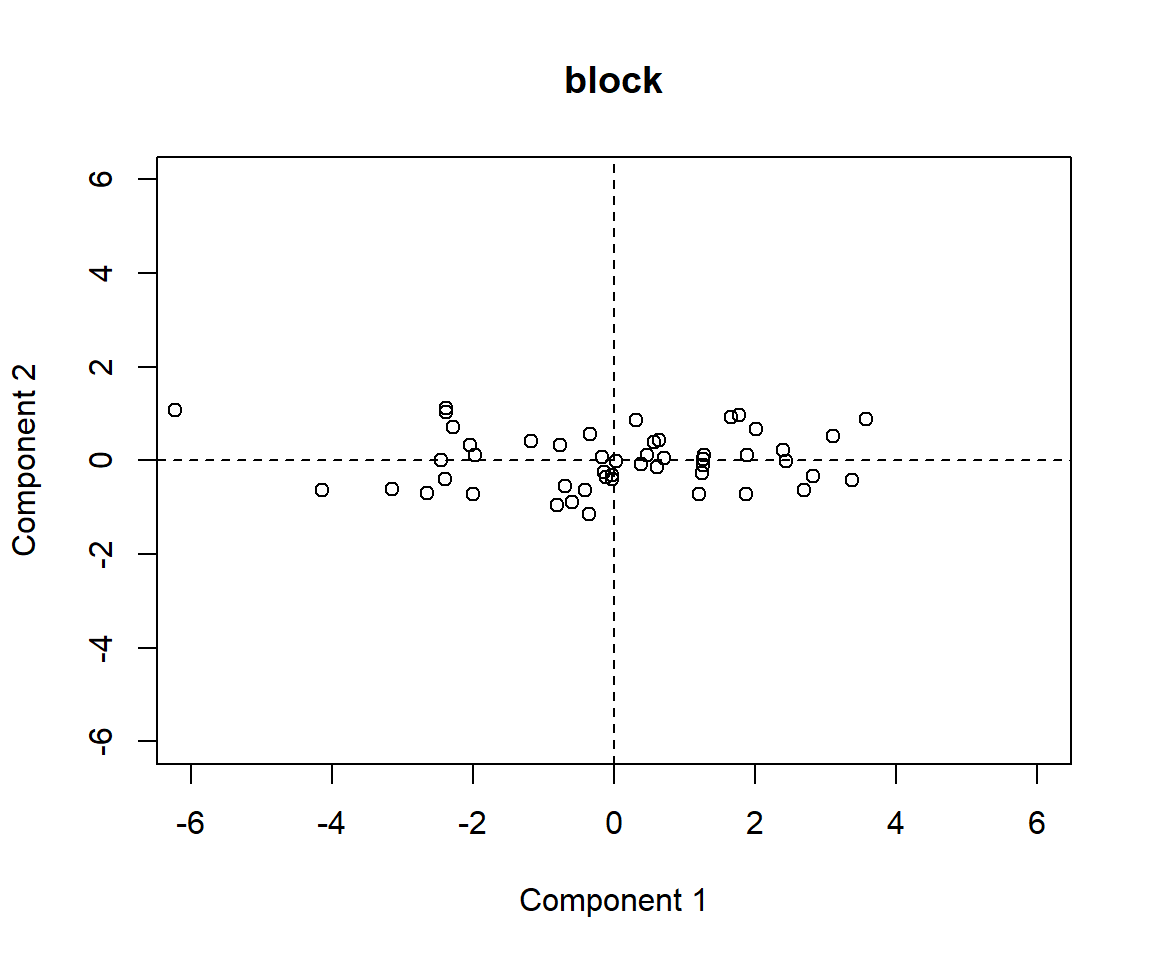``plot(fit111, v="score", axes = 2:3, plottype="scatter")``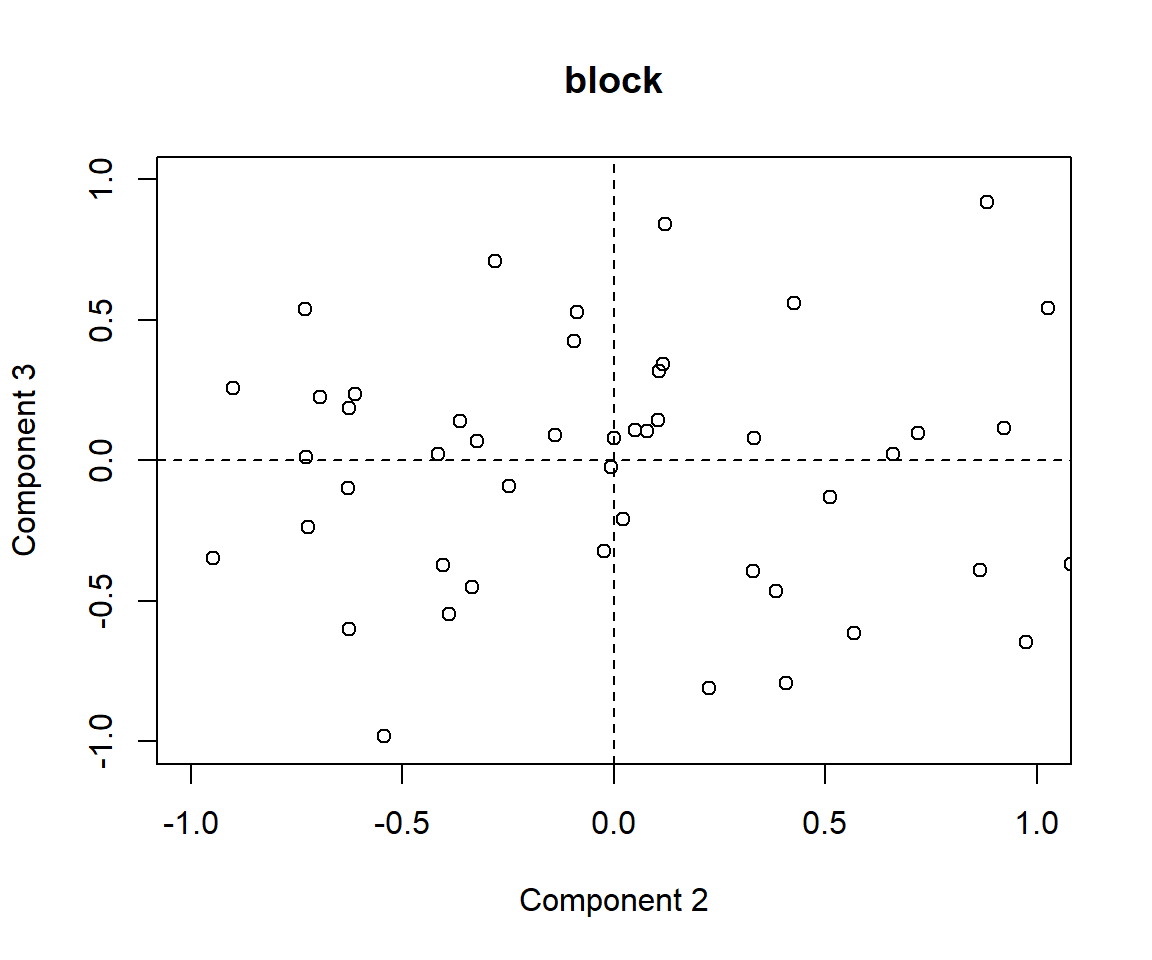When the argument `v` was specified as “cpev”, the cummulative eigenvalues are plotted.

``````par(mfrow=c(1,1))
plot(fit111, v="cpev")``````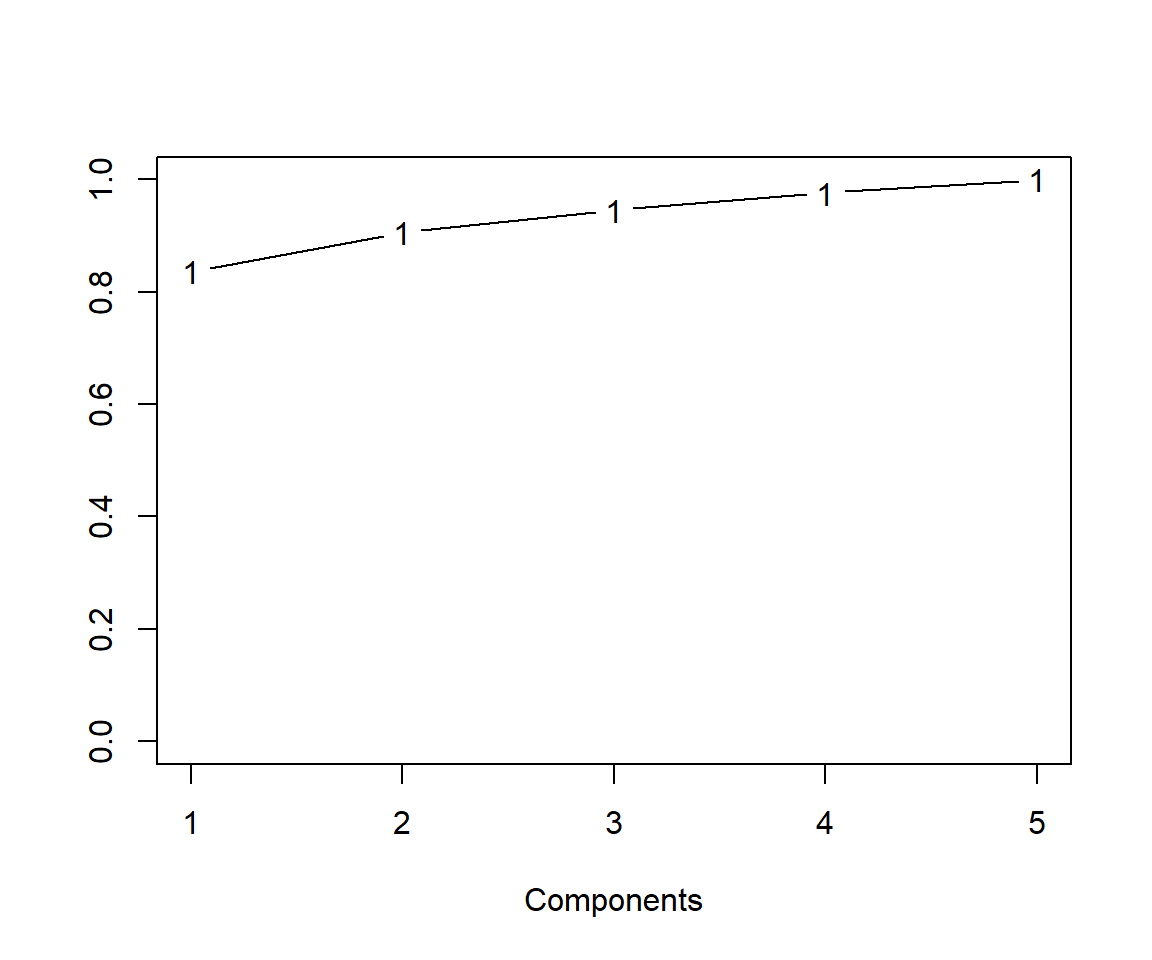### Other functions in R for PCA

There is the R function prcomp to implement PCA.

``(fit1112 = prcomp(X1, scale=TRUE))``
``````## Standard deviations (1, .., p=5):
##  2.0446732 0.5899513 0.4458638 0.3926788 0.3439156
##
## Rotation (n x k) = (5 x 5):
##             PC1         PC2         PC3           PC4         PC5
## [1,] -0.4309746  0.74172462 -0.03722419  1.351296e-01 -0.49442882
## [2,] -0.4483141 -0.31171881  0.63044575  5.510830e-01  0.02629751
## [3,] -0.4601629  0.19547669 -0.38616901  1.416349e-01  0.76213651
## [4,] -0.4392701 -0.55816074 -0.57114566 -4.296727e-05 -0.41144993
## [5,] -0.4566918 -0.05405032  0.35470924 -8.111640e-01  0.06859643``````
``summary(fit1112)``
``````## Importance of components:
##                           PC1     PC2     PC3     PC4     PC5
## Standard deviation     2.0447 0.58995 0.44586 0.39268 0.34392
## Proportion of Variance 0.8361 0.06961 0.03976 0.03084 0.02366
## Cumulative Proportion  0.8361 0.90575 0.94551 0.97634 1.00000``````
``biplot(fit1112)``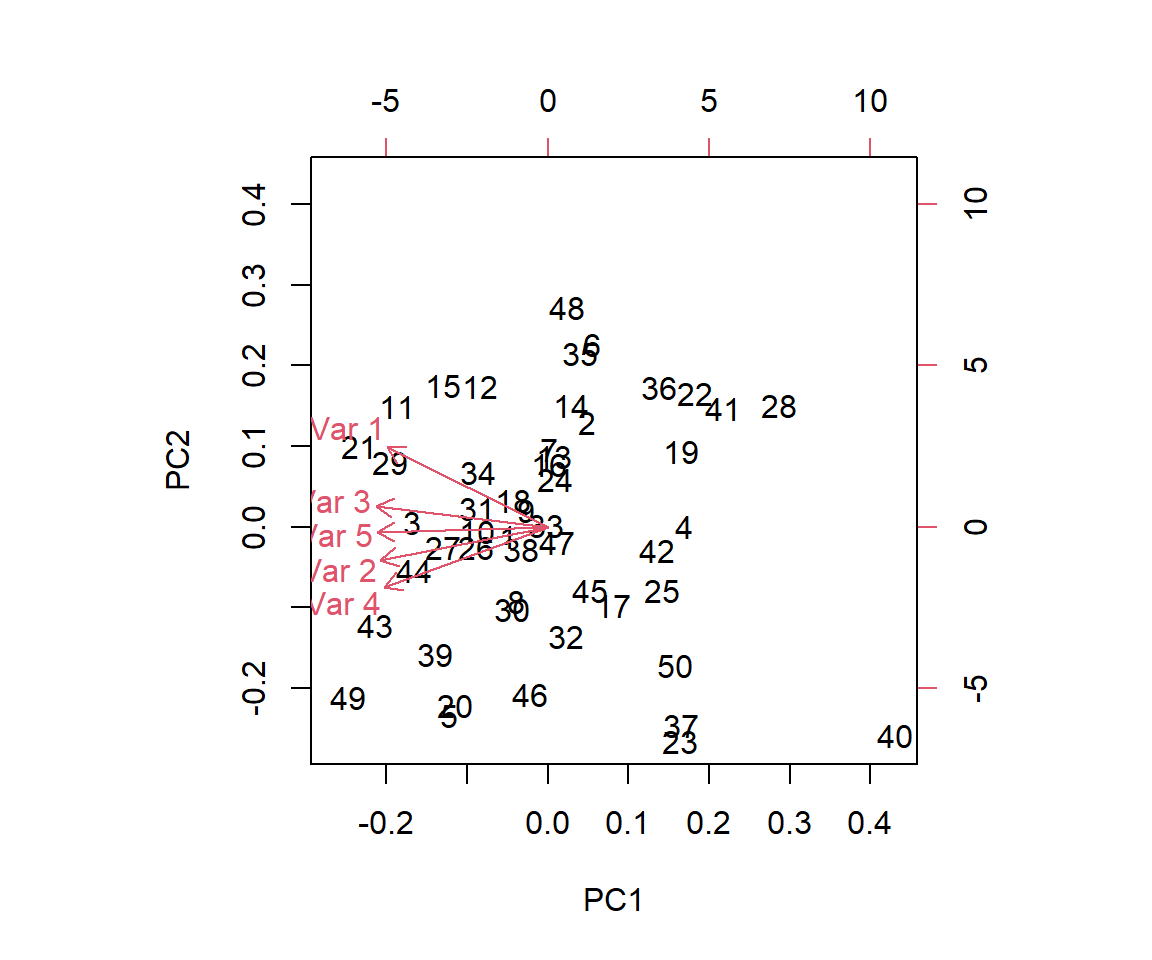The `ggfortify` package is also available for the PCA plot.

### Sparse PCA

If `lambdaX` (>0) is specified, a sparse principal component analysis is implemented.

``(fit112 = msma(X1, comp=5, lambdaX=0.1))``
``````## Call:
## msma.default(X = X1, comp = 5, lambdaX = 0.1)
##
## Numbers of non-zeros for X:
##        comp1 comp2 comp3 comp4 comp5
## block1     5     4     4     5     4
##
## Numbers of non-zeros for X super:
## comp1 comp2 comp3 comp4 comp5
##     1     1     1     1     1``````
``````par(mfrow=c(1,2))
plot(fit112, axes = 1, plottype="bar")
plot(fit112, axes = 2, plottype="bar")``````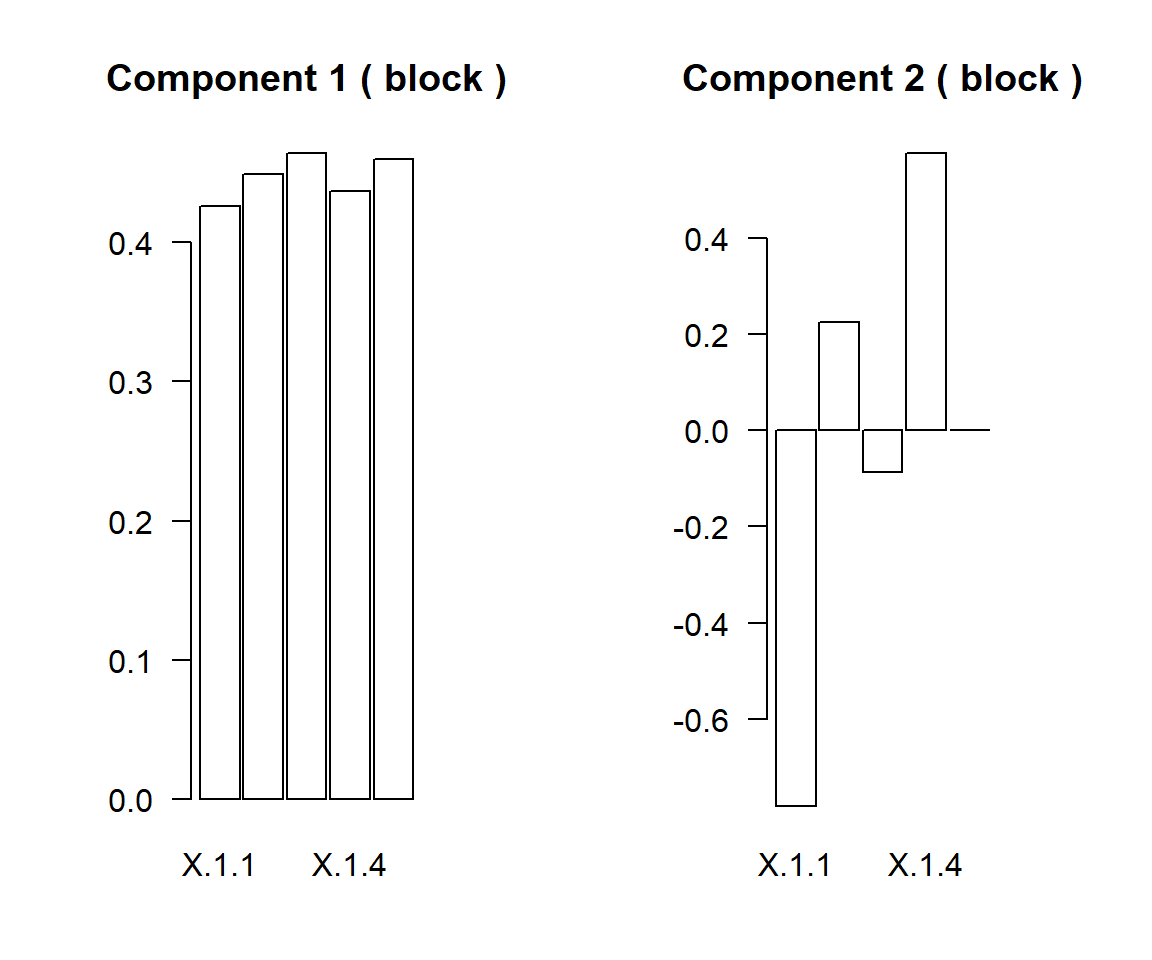### Supervised Sparse PCA

The outcome Z is generated.

``set.seed(1); Z = rbinom(50, 1, 0.5)``

If the outcome Z is specified, a supervised sparse principal component analysis is implemented.

``(fit113 = msma(X1, Z=Z, comp=5, lambdaX=0.02))``
``````## Call:
## msma.default(X = X1, Z = Z, comp = 5, lambdaX = 0.02)
##
## Numbers of non-zeros for X:
##        comp1 comp2 comp3 comp4 comp5
## block1     5     5     5     4     5
##
## Numbers of non-zeros for X super:
## comp1 comp2 comp3 comp4 comp5
##     1     1     1     1     1``````
``````par(mfrow=c(1,2))
plot(fit113, axes = 1, plottype="bar")
plot(fit113, axes = 2, plottype="bar")``````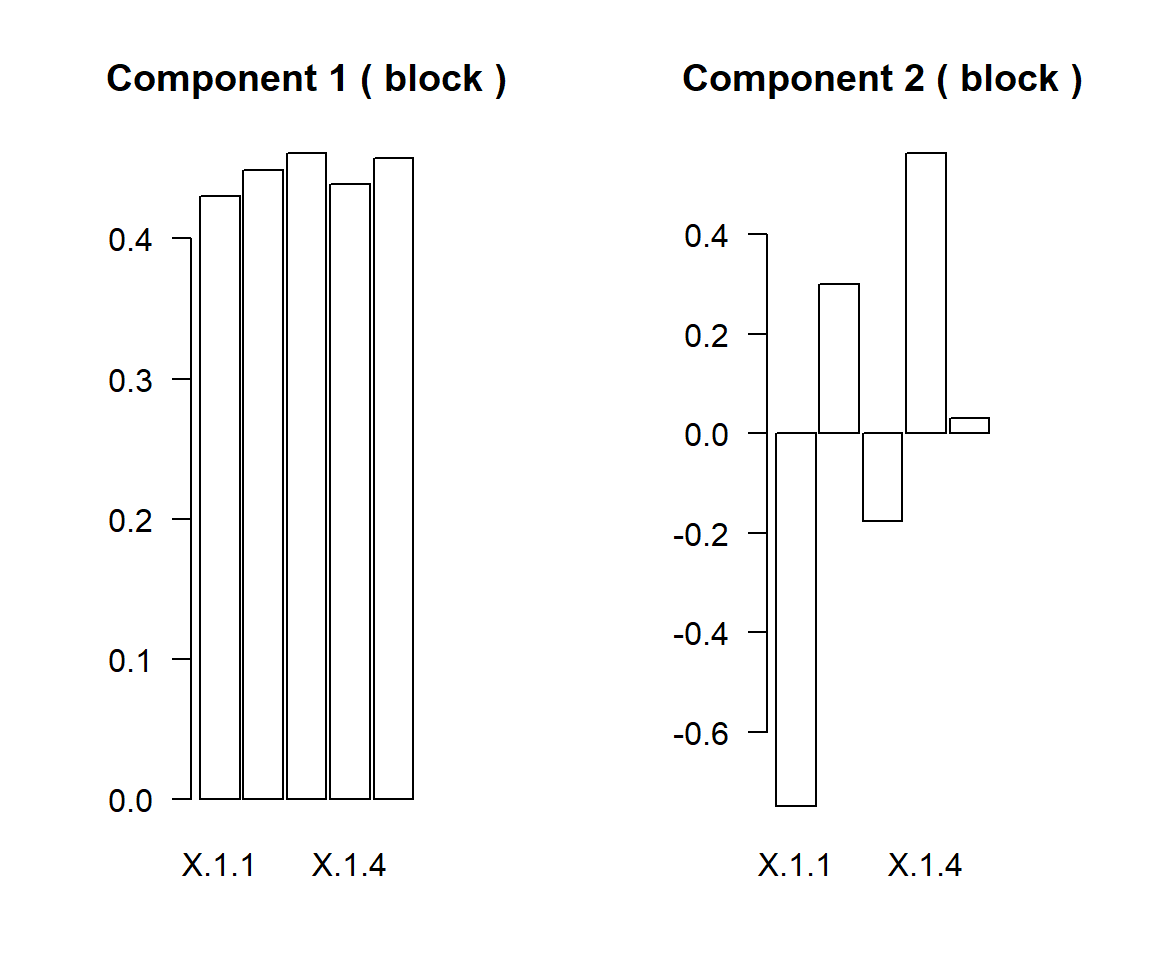## Partial Least Squres (PLS)

If the another input Y1 is specified, a partial least squres is implemented.

``(fit121 = msma(X1, Y1, comp=2))``
``````## Call:
## msma.default(X = X1, Y = Y1, comp = 2)
##
## Numbers of non-zeros for X:
##        comp1 comp2
## block1     5     5
##
## Numbers of non-zeros for X super:
## comp1 comp2
##     1     1
##
## Numbers of non-zeros for Y:
##        comp1 comp2
## block1     5     5
##
## Numbers of non-zeros for Y super:
## comp1 comp2
##     1     1``````

The component number is specified by the argument `axes`. When the argument `XY` was specified as “XY”, the scatter plots for Y score against X score are plotted.

``````par(mfrow=c(1,2))
plot(fit121, axes = 1, XY="XY")
plot(fit121, axes = 2, XY="XY")``````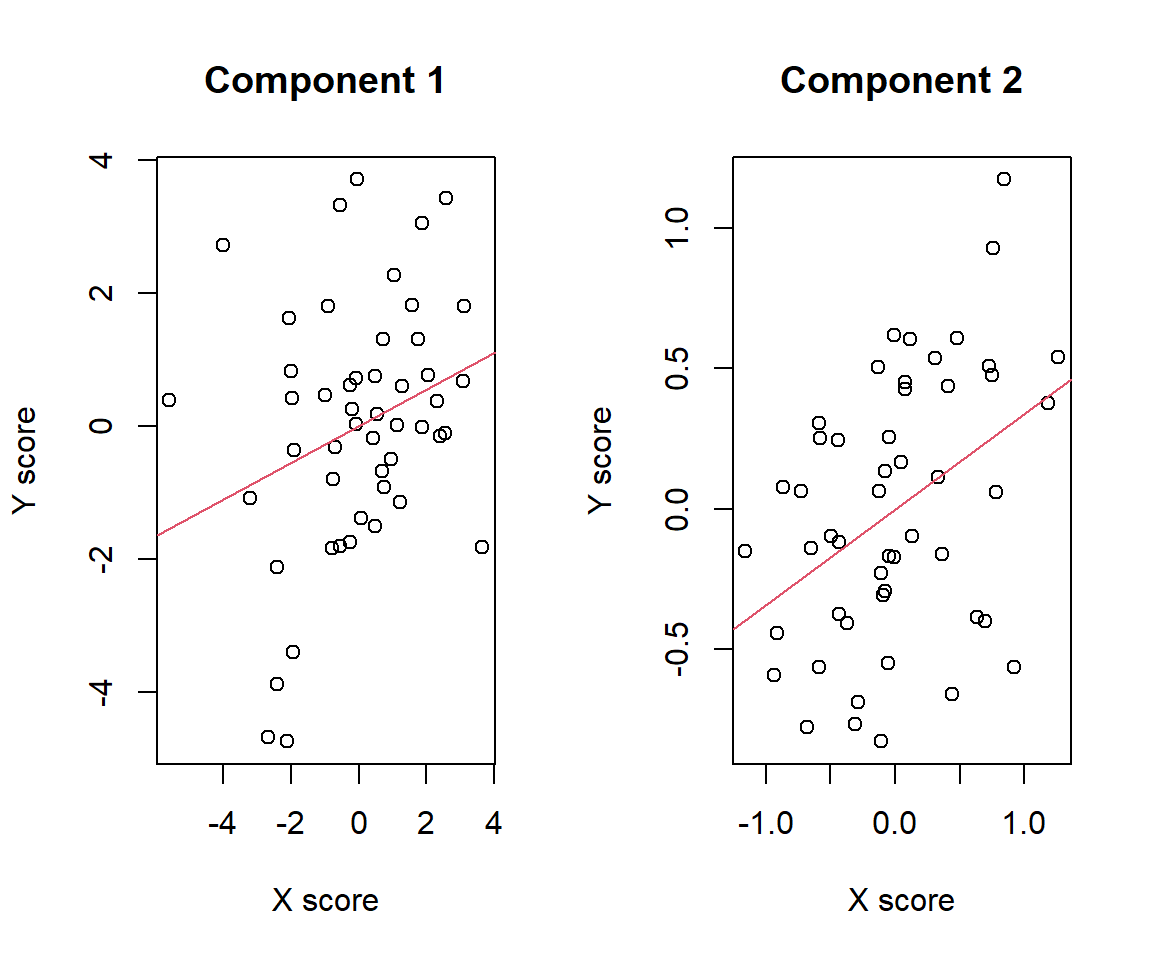### Sparse PLS

If `lambdaX` and `lambdaY` are specified, a sparse PLS is implemented.

``(fit122 = msma(X1, Y1, comp=2, lambdaX=0.5, lambdaY=0.5))``
``````## Call:
## msma.default(X = X1, Y = Y1, comp = 2, lambdaX = 0.5, lambdaY = 0.5)
##
## Numbers of non-zeros for X:
##        comp1 comp2
## block1     2     2
##
## Numbers of non-zeros for X super:
## comp1 comp2
##     1     1
##
## Numbers of non-zeros for Y:
##        comp1 comp2
## block1     2     2
##
## Numbers of non-zeros for Y super:
## comp1 comp2
##     1     1``````
``````par(mfrow=c(1,2))
plot(fit122, axes = 1, XY="XY")
plot(fit122, axes = 2, XY="XY")``````